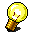School-Based Intervention Idea from www.interventioncentral.orgFocus: MATHEMATICS Applied Problems: Improving Performance Through a 4-Step Problem-Solving Approach  (Pólya, 1957; Williams, 2003) Students can consistently perform better on applied math problems if they follow an efficient 4-step plan of understanding the problem, devising a plan, carrying out the plan, and looking back. (1) UNDERSTAND THE PROBLEM. To fully grasp the problem, the student may restate the problem in his or her own words, note key information, and identify missing information. (2) DEVISE A PLAN. In mapping out a strategy to solve the problem, the student may make a table, draw a diagram, or translate the verbal problem into an equation. (3) CARRY OUT THE PLAN. The student implements the steps in the plan, showing work and checking work for each step. (4) LOOK BACK. The student checks the results. If the answer is written as an equation, the student puts the results in words and checks whether the answer addresses the question posed in the original word problem. References Pólya, G. (1957). How to solve it (2nd ed.). Princeton University Press: Princeton, N.J. Williams, K. M. (2003). Writing about the problem solving process to improve problem-solving performance. Mathematics Teacher, 96(3), 185-187. Copyright ©2021 Jim Wright